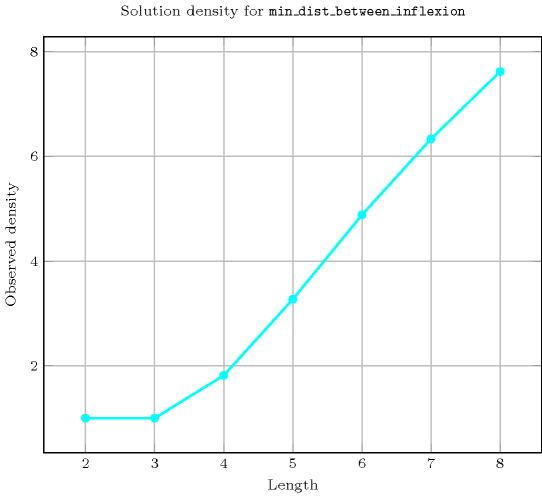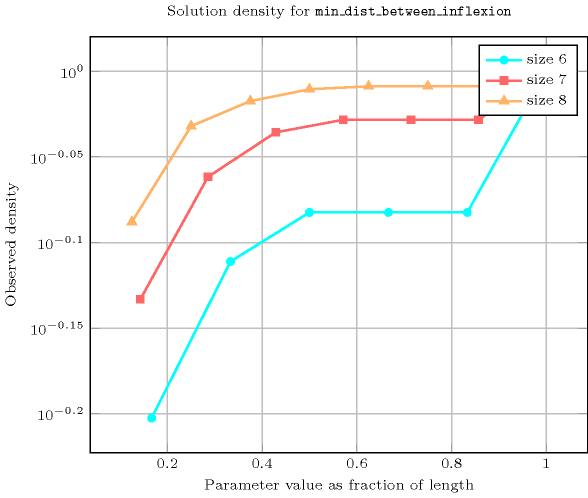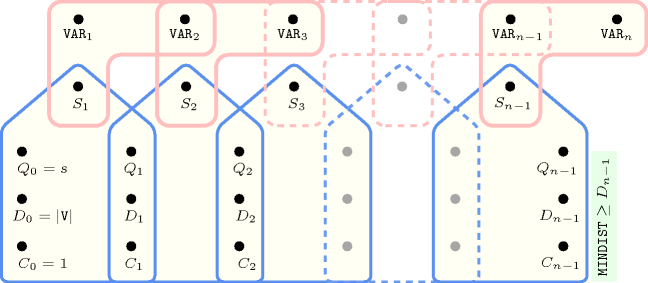## 5.253. min_dist_between_inflexion

Origin
Constraint

$\mathrm{𝚖𝚒𝚗}_\mathrm{𝚍𝚒𝚜𝚝}_\mathrm{𝚋𝚎𝚝𝚠𝚎𝚎𝚗}_\mathrm{𝚒𝚗𝚏𝚕𝚎𝚡𝚒𝚘𝚗}\left(\mathrm{𝙼𝙸𝙽𝙳𝙸𝚂𝚃},\mathrm{𝚅𝙰𝚁𝙸𝙰𝙱𝙻𝙴𝚂}\right)$

Arguments
 $\mathrm{𝙼𝙸𝙽𝙳𝙸𝚂𝚃}$ $\mathrm{𝚒𝚗𝚝}$ $\mathrm{𝚅𝙰𝚁𝙸𝙰𝙱𝙻𝙴𝚂}$ $\mathrm{𝚌𝚘𝚕𝚕𝚎𝚌𝚝𝚒𝚘𝚗}\left(\mathrm{𝚟𝚊𝚛}-\mathrm{𝚍𝚟𝚊𝚛}\right)$
Restrictions
 $\mathrm{𝙼𝙸𝙽𝙳𝙸𝚂𝚃}\ge 0$ $\mathrm{𝙼𝙸𝙽𝙳𝙸𝚂𝚃}\le |\mathrm{𝚅𝙰𝚁𝙸𝙰𝙱𝙻𝙴𝚂}|$ $\mathrm{𝚛𝚎𝚚𝚞𝚒𝚛𝚎𝚍}$$\left(\mathrm{𝚅𝙰𝚁𝙸𝙰𝙱𝙻𝙴𝚂},\mathrm{𝚟𝚊𝚛}\right)$
Purpose

Given an integer value $\mathrm{𝙼𝙸𝙽𝙳𝙸𝚂𝚃}$ and a sequence of variables $\mathrm{𝚅𝙰𝚁𝙸𝙰𝙱𝙻𝙴𝚂}$ enforce $\mathrm{𝙼𝙸𝙽𝙳𝙸𝚂𝚃}$ to be greater than or equal to the smallest distance between two consecutive inflexions in the sequence $\mathrm{𝚅𝙰𝚁𝙸𝙰𝙱𝙻𝙴𝚂}$, or to $|\mathrm{𝚅𝙰𝚁𝙸𝙰𝙱𝙻𝙴𝚂}|$ if no more than one inflexion exists.

An inflexion of a sequence of variables $\mathrm{𝚅𝙰𝚁𝙸𝙰𝙱𝙻𝙴𝚂}$ is a set of consecutive variables ${V}_{i},{V}_{i+1},\cdots ,{V}_{j-1},{V}_{j}$ ($i+1) such that one of the following conditions holds:

• ${V}_{i}<{V}_{i+1}\wedge {V}_{i+1}=\cdots ={V}_{j-1}\wedge {V}_{j-1}>{V}_{j}$,

• ${V}_{i}>{V}_{i+1}\wedge {V}_{i+1}=\cdots ={V}_{j-1}\wedge {V}_{j-1}<{V}_{j}$.

In this context, the index $j$ is the position of the inflexion (i.e., the first instant when the inflexion is discovered when scanning the sequence of variables $\mathrm{𝚅𝙰𝚁𝙸𝙰𝙱𝙻𝙴𝚂}$ from left to right. The distance between two consecutive inflexions is the absolute value of the difference of their corresponding positions.

Example
$\left(2,〈2,2,3,3,2,2,1,4,4,3〉\right)$

Figure 5.253.1 shows the three inflexions associated with the sequence 2, 2, 3, 3, 2, 2, 1, 4, 4, 3 and their respective positions 5, 8 and 10 in red. The $\mathrm{𝚖𝚒𝚗}_\mathrm{𝚍𝚒𝚜𝚝}_\mathrm{𝚋𝚎𝚝𝚠𝚎𝚎𝚗}_\mathrm{𝚒𝚗𝚏𝚕𝚎𝚡𝚒𝚘𝚗}$ constraint holds since its first argument $\mathrm{𝙼𝙸𝙽𝙳𝙸𝚂𝚃}=2$ is greater than or equal to the smallest distance 2 between two consecutive inflexions of the sequence of variables $\mathrm{𝚅𝙰𝚁𝙸𝙰𝙱𝙻𝙴𝚂}$.

##### Figure 5.253.1. Illustration of the Example slot: a sequence of ten variables ${V}_{1}$, ${V}_{2}$, ${V}_{3}$, ${V}_{4}$, ${V}_{5}$, ${V}_{6}$, ${V}_{7}$, ${V}_{8}$, ${V}_{9}$, ${V}_{10}$ respectively fixed to values 2, 2, 3, 3, 2, 2, 1, 4, 4, 3 and its three inflexions, two peaks and one valley; each red point denotes an instant where a new inflexion is discovered while scanning the sequence from left to right; as shown by the rightmost arrow, the minimum distance between two consecutive inflexions is equal to 2.Typical
 $\mathrm{𝙼𝙸𝙽𝙳𝙸𝚂𝚃}>1$ $|\mathrm{𝚅𝙰𝚁𝙸𝙰𝙱𝙻𝙴𝚂}|>3$ $\mathrm{𝚛𝚊𝚗𝚐𝚎}$$\left(\mathrm{𝚅𝙰𝚁𝙸𝙰𝙱𝙻𝙴𝚂}.\mathrm{𝚟𝚊𝚛}\right)>1$
Symmetries
• Items of $\mathrm{𝚅𝙰𝚁𝙸𝙰𝙱𝙻𝙴𝚂}$ can be reversed.

• One and the same constant can be added to the $\mathrm{𝚟𝚊𝚛}$ attribute of all items of $\mathrm{𝚅𝙰𝚁𝙸𝙰𝙱𝙻𝙴𝚂}$.

Counting
 Length ($n$) 2 3 4 5 6 7 8 Solutions 9 64 1135 25444 574483 13287476 328156407

Number of solutions for $\mathrm{𝚖𝚒𝚗}_\mathrm{𝚍𝚒𝚜𝚝}_\mathrm{𝚋𝚎𝚝𝚠𝚎𝚎𝚗}_\mathrm{𝚒𝚗𝚏𝚕𝚎𝚡𝚒𝚘𝚗}$: domains $0..n$Length ($n$)2345678
Total96411352544457448313287476328156407
 Parameter value

1--170359873794154351235152278
29-170469091098181976439992562
3-64170469097314193201241360676
4--625469097314196501242025560
5---777697314196501242192870
6----117649196501242192870
7-----209715242192870
8------43046721

Solution count for $\mathrm{𝚖𝚒𝚗}_\mathrm{𝚍𝚒𝚜𝚝}_\mathrm{𝚋𝚎𝚝𝚠𝚎𝚎𝚗}_\mathrm{𝚒𝚗𝚏𝚕𝚎𝚡𝚒𝚘𝚗}$: domains $0..n$Figure 5.253.2 depicts the automaton associated with the $\mathrm{𝚖𝚒𝚗}_\mathrm{𝚍𝚒𝚜𝚝}_\mathrm{𝚋𝚎𝚝𝚠𝚎𝚎𝚗}_\mathrm{𝚒𝚗𝚏𝚕𝚎𝚡𝚒𝚘𝚗}$ constraint.
##### Figure 5.253.2. Automaton of the $\mathrm{𝚖𝚒𝚗}_\mathrm{𝚍𝚒𝚜𝚝}_\mathrm{𝚋𝚎𝚝𝚠𝚎𝚎𝚗}_\mathrm{𝚒𝚗𝚏𝚕𝚎𝚡𝚒𝚘𝚗}$ constraint (state $s$ means that we are in stationary mode, state $i0$ means that we are in increasing mode and that we did not yet found any inflexion, state $d0$ means that we are in decreasing mode and that we did not yet found any inflexion, state $i1$ means that we are in increasing mode and that we already found at least one inflexion, state $d1$ means that we are in decreasing mode and that we already found at least one inflexion, the minimum distance between two consecutive inflexions is updated each time we switch from $i1$ to $d1$ mode – or conversely from $d1$ to $i1$ mode – and the counter $D$ is updated accordingly)##### Figure 5.253.3. Hypergraph of the reformulation corresponding to the automaton of the $\mathrm{𝚖𝚒𝚗}_\mathrm{𝚍𝚒𝚜𝚝}_\mathrm{𝚋𝚎𝚝𝚠𝚎𝚎𝚗}_\mathrm{𝚒𝚗𝚏𝚕𝚎𝚡𝚒𝚘𝚗}$ constraint where $𝚅$ is a shortcut for $\mathrm{𝚅𝙰𝚁𝙸𝙰𝙱𝙻𝙴𝚂}$ (since all states of the automaton are accepting there is no restriction on the last variable ${Q}_{n-1}$)